# Astroid

(diff) ← Older revision | Latest revision (diff) | Newer revision → (diff)

A plane algebraic curve of order six, described by a pointon a circle of radiusrolling on the inside of a circle of radius; a hypocycloid with module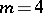. Its equation in orthogonal Cartesian coordinates is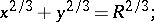and a parametric representation is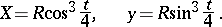Figure: a013540a

There are four cusps (see Fig.). The length of the arc from the pointisThe length of the entire curve is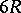. The radius of curvature isThe area bounded by the curve is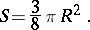The astroid is the envelope of a family of segments of constant length, the ends of which are located on two mutually perpendicular straight lines. This property of the astroid is connected with one of its generalizations — the so-called oblique astroid, which is the envelope of the segments of constant length with their ends located on two straight lines intersecting at an arbitrary angle.

How to Cite This Entry:
Astroid. Encyclopedia of Mathematics. URL: http://encyclopediaofmath.org/index.php?title=Astroid&oldid=13095
This article was adapted from an original article by D.D. Sokolov (originator), which appeared in Encyclopedia of Mathematics - ISBN 1402006098. See original article